# 面试官：请手写一个简易的单链表​

WilliamGates

0收藏

### 1、链表是什么？具备哪些基本特征？

• 物理存储非连续、逻辑通过指针实现顺序性,其示例图如下所示：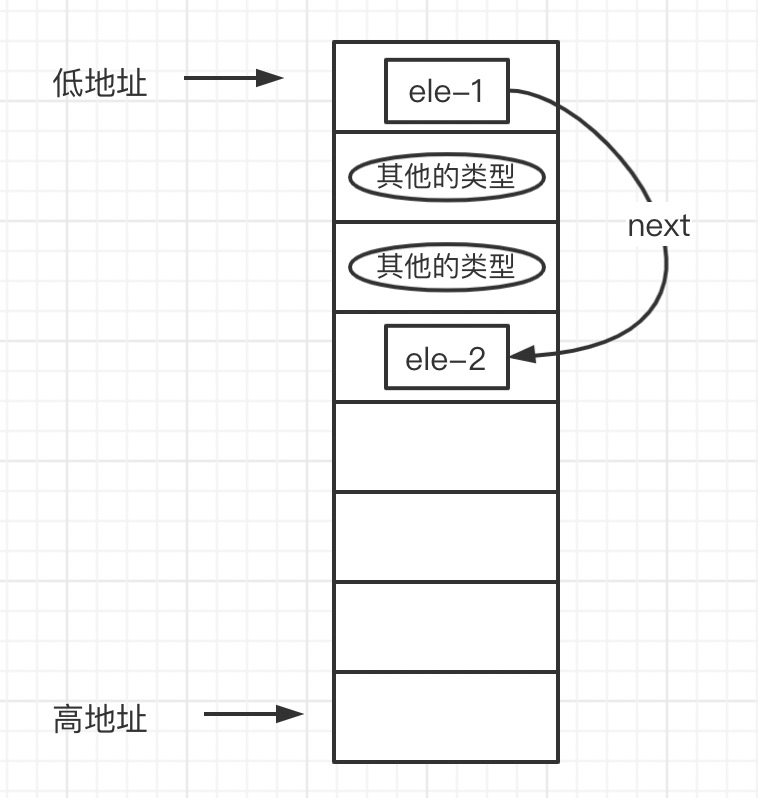• 数据结构分为指针域和数据域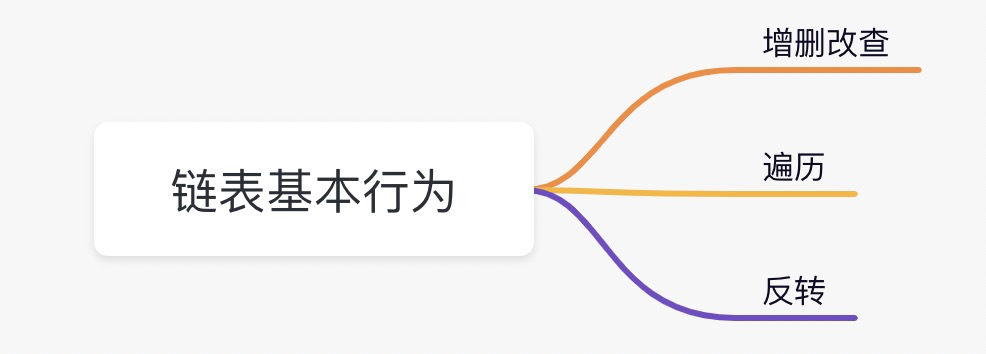### 2、图解与代码实现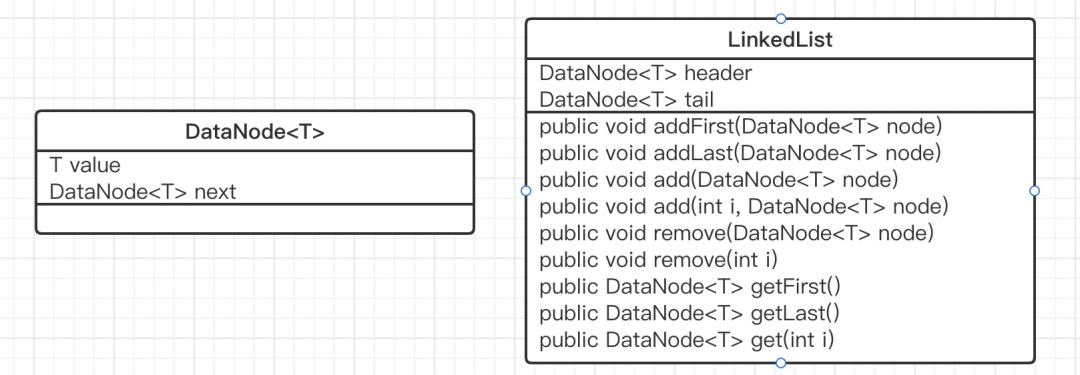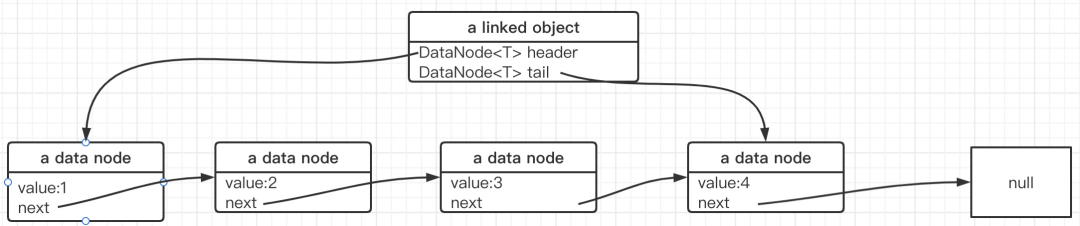#### 2.1 基础代码

``````public class LinkedList<T> {

/** 指针域*/
// 头节点
// 从节点
private DataNode<T> tail;

private int size;

/**
* 数据节点
* @param <T>
*/
private class DataNode<T> {
public T value;
public DataNode<T> next;

public DataNode(T value){
this.value = value;
}
}
}``````

#### 2.2 指定下标插入节点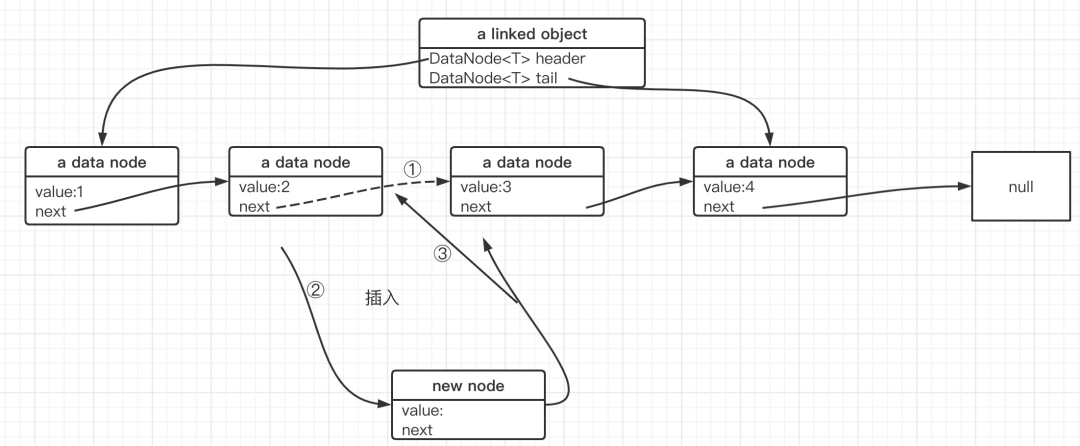``````public void add(int index, T value){
if(index >= size) {
throw new ArrayIndexOutOfBoundsException();
}
DataNode<T> node = new DataNode<>(value);
DataNode<T> prev = get(index);

DataNode<T> tmp = prev.next;  // @1
prev.next = node;             // @2
node.next = tmp;              // @3
}``````

• @1：首先创建一个临时节点，用于暂存前驱节点的next
• @2：前驱节点的next指针重新指向待插入的节点
• @3：新节点的next指针指向原前驱节点的next指针

``````node.next = prev.next;
prev.next = node;``````

#### 2.3 移除指定下标处节点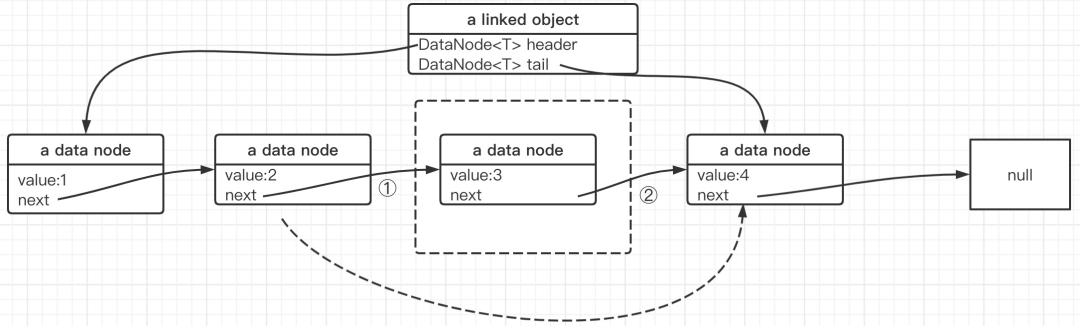``````public T remove(int index){
if(index >= size) {
throw new ArrayIndexOutOfBoundsException();
}

DataNode<T> pre = get(index - 1 );
DataNode<T> cur = pre.next;

pre.next = cur.next;
// help GC
cur.next = null;

size --;
return cur.value;
}``````

#### 2.4 单链表反转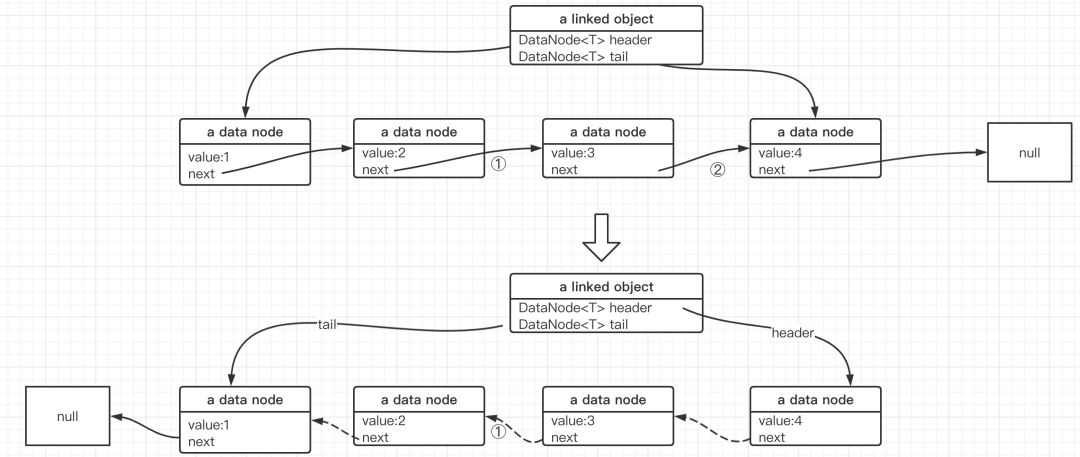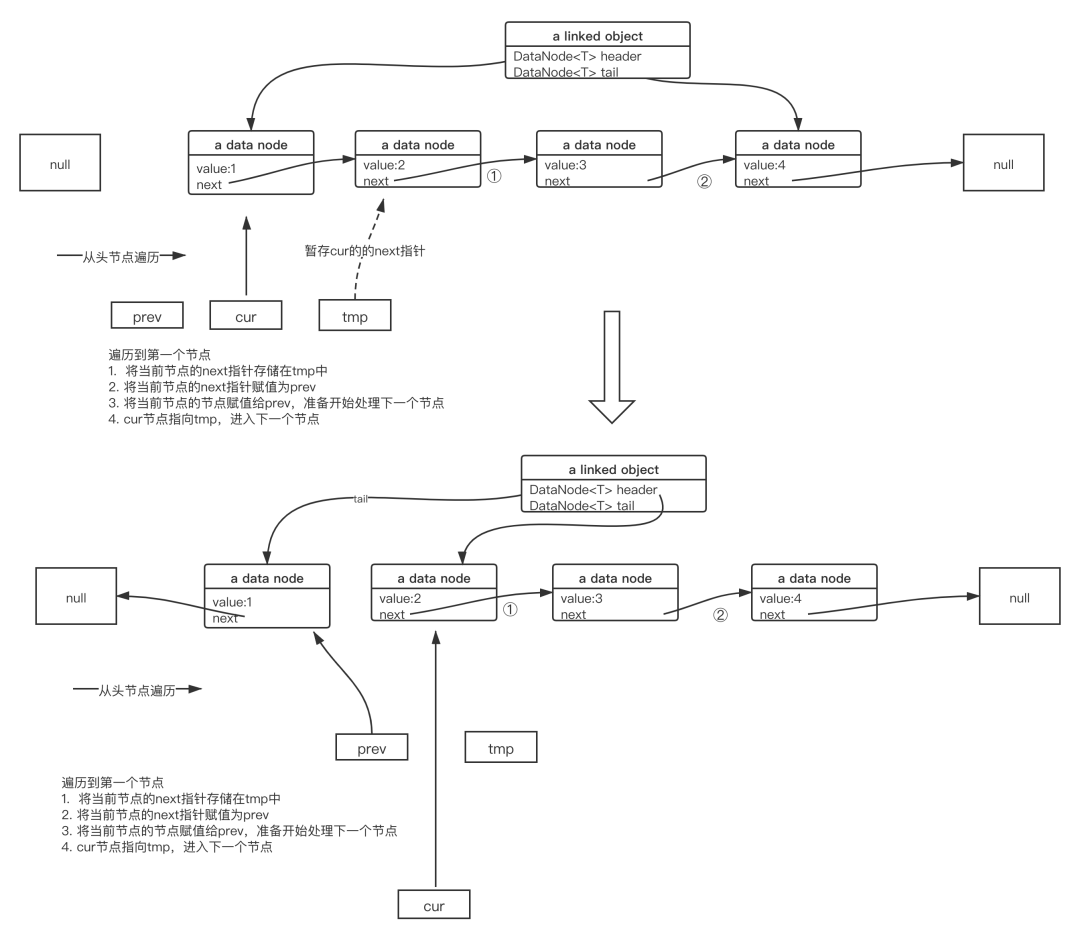``````public void reverse(){
// 从头节点开始遍历
// 记录当前节点到 prev 前驱节点
DataNode<T> cur_prev = null;
// 暂存当前节点到next指针
DataNode<T> cur_next = null;

// 从当前节点开始遍历，直接到尾部
while(cur != null) {
//反转之前，先暂存相关节点
cur_next = cur.next;
cur.next = cur_prev;
cur_prev = cur;
// 继续遍历下一个节点
cur = cur_next;
}

tail = tmp;
}``````

### 3、常见面试题

#### 3.1 链表与数组的区别

• 内存布局
• 插入性能
• 查找性能

##### 3.1.2 插入性能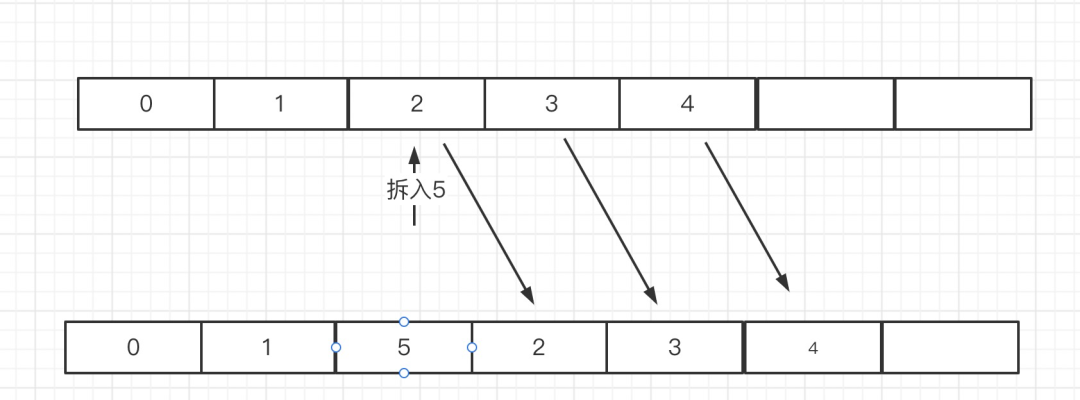##### 3.1.3 查找性能帖子
视频
声望
粉丝
最近发布
社区精华内容